# Sample Midterm¶

Things to know for the midterm

• Bayes' rule, sum and product rules of probability, expectations
• Conditioning, normalization, marginalization
• Exponential family distributions, deriving their sufficient statistics and maximum likelihood
• Relationship between graphical model and factorization of joint distribution
• Determining conditional independence
• Variable Elimination and complexity of inference

## Question 1¶

Recall that the definition of an exponential family model is:

$f(x | \eta) = h(x)g(\eta)\exp(\eta^TT(x))$

where

• $$\eta$$ are the parameters
• $$T(x)$$ are the sufficient statistics
• $$h(x)$$ is the base measure
• $$g(\eta)$$ is the normalizing constant

Consider the univariate Gaussian, with mean $$\mu$$ and precision $$\lambda = \frac{1}{\sigma^2}$$

$p(D | \mu \lambda) = \prod^N_{i=1}(\frac{\lambda}{2\pi})^{\frac{1}{2}}\exp(-\frac{\lambda}{2}(x_i - \mu)^2)$

What are $$\eta$$ and $$T(x)$$ for this distribution when represented in exponential family form?

Solution

Start by expanding the terms in the exponent

$= \prod^N_{i=1}(\frac{\lambda}{2\pi})^{\frac{1}{2}} \exp(\sum_{i=1}^N -\frac{\lambda}{2}x_i^2 + \lambda u x_i - \frac{\lambda}{2}\mu^2) \\$

from here, we can rearrange the exponent into $$\eta^TT(x)$$

$= \prod^N_{i=1}(\frac{\lambda}{2\pi})^{\frac{1}{2}}\exp(\sum_{i=1}^N - \frac{\lambda}{2}\mu^2)\exp(\begin{bmatrix}\lambda \mu & -\frac{\lambda}{2} \end{bmatrix} \begin{bmatrix}\sum_{i=1}^N x_i\\ \sum_{i=1}^N x_i^2\end{bmatrix}$

where

• $$\eta^T = \begin{bmatrix}\lambda \mu & -\frac{\lambda}{2}\end{bmatrix}$$
• $$T(x) = \begin{bmatrix}\sum_{i=1}^N x_i\\ \sum_{i=1}^N x_i^2\end{bmatrix}$$

## Question 2¶

Consider the following directed graphical model: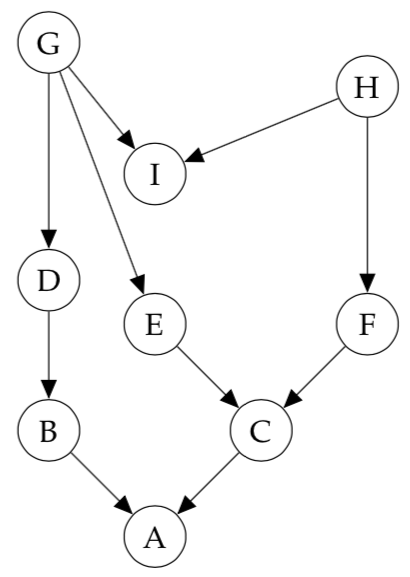a) List all variables that are independent of $$A$$ given evidence on $$B$$

Solution

By Bayes' Balls, no variables are conditionally independent of $$A$$ given evidence on $$B$$.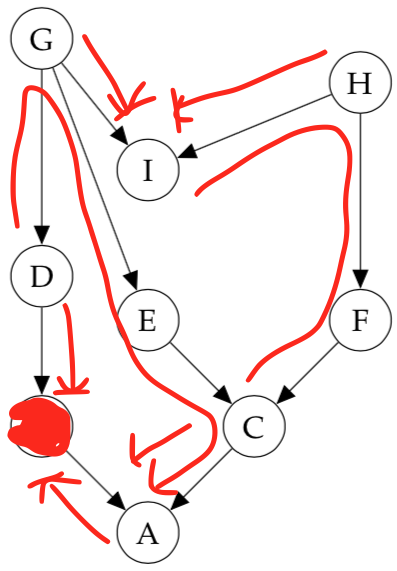b) Write down the factorized normalized joint distribution that this graphical model represents.

Solution
$p(A, ..., I) = p(A | B, C)P(B | D)P(C | E, F)P(D | G)P(E | G)P(F | H)P(G)P(H)P(I | G, H)$

c) If each node is a discrete random variable in $$\{1, ..., K\}$$ how many distinct joint states can the model take? That is, how many different configurations can the variables in this model be set?

Solution

For each node (random variable) there is $$k$$ states. There are $$k^n$$ possible configurations where $$k$$ is the number of states and $$n$$ the number of nodes ($$x_i$$)

$\therefore \text{number of possible configurations} = k^9$

## Question 3¶

Consider the Hidden Markov Model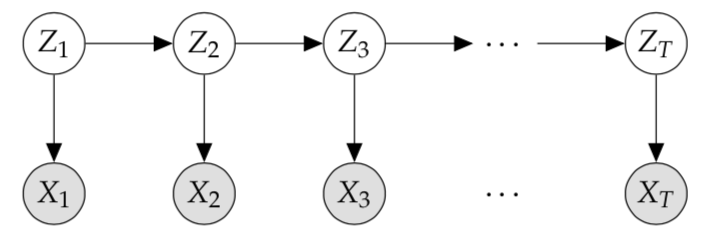Assume you are able to sample from these conditional distributions, i.e.

$x_i \sim p(X_i \ | \ \text{parents of } X_i)$

Write down a step-by-step process to produce a sample observation from this model, i.e. $$(x_1, x_2, x_3, ..., x_T)$$ in terms of samples from the individual factors.

Solution

We want to sample a sequence of observations $$x_1, x_2, x_3, ..., x_T$$ from the model according to

$x_{1:T} = \prod_{t=1}^T p(X_t \ | \ \text{parents of } X_t)$

since observations $$x_t$$ are independent of one another. Notice that this forms a chain, with probability

$p(x_{1:T}) \sim \bigg [ \prod_{t=1}^T p(X_t | z_t) \bigg ] \bigg [ p(z_1) \prod_{t=2}^T p(Z_t | z_{t-1}) \bigg ]$

Step-by-step

1. Start with $$t=1$$
2. Sample $$z_t$$ according to $$z_t \sim p(z_1) \prod_{i=t}^{t + 1} p(Z_i | z_{i-1})$$
3. Given the sampled $$z_t$$, sample $$x_t$$ according to $$x_t \sim \ p(X_t | z_t)$$
4. Increment $$t$$ by 1
5. Repeat steps 2-4 until $$t=T$$

## Question 4¶

Consider the graphical model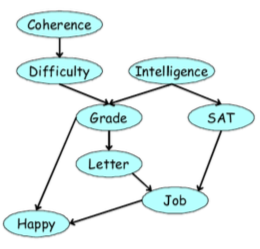a) Write down the factorization of the joint distribution given by this graphical model.

Solution
$p(C, D, I, G, S, L, H, J) = p(C)p(D|C)p(I)p(G | D, I)p(L | G)P(S | I)p(J | S, L)p(H | J, G)$

b) Use the Variable Elimination algorithm to find $$p(J)$$ with the ordering $$\prec \{G, I, S, L, H, C, D\}$$. What is the complexity of the VE algorithm with this ordering?

Solution
\begin{aligned} p(J) &= \sum_D \sum_C \phi(C)\phi(C,D) \sum_H \sum_L \sum_S \phi(J,L,S)\sum_I \phi(S,I)\phi(I) \underbrace{\sum_G\phi(G,D,I)\phi(L,G) \phi(H,G,J)}_{\tau(D,I,L,H,J), N_G = 6}\\ &= \sum_D \sum_C \phi(C)\phi(C,D) \sum_H \sum_L \sum_S \phi(J,L,S)\underbrace{\sum_I \phi(S,I)\phi(I) \tau(D,I,L,H,J)}_{\tau(D,L,H,J,S), N_I = 6}\\ &= \sum_D \sum_C \phi(C)\phi(C,D) \sum_H \sum_L \underbrace{\sum_S \phi(J,L,S)\tau(D,L,H,J,S)}_{\tau(D,L,H,J), N_S = 5}\\ &= \sum_D \sum_C \phi(C)\phi(C,D) \sum_H \underbrace{\sum_L \tau(D,L,H,J)}_{\tau(D,H,J), N_L = 4}\\ &= \sum_D \sum_C \phi(C)\phi(C,D) \underbrace{\sum_H \tau(D,H,J)}_{\tau(D,J), N_H = 3}\\ &= \sum_D \tau(D,J) \underbrace{\sum_C \phi(C)\phi(C,D)}_{\tau(D), N_C = 2}\\ &= \underbrace{\sum_D\tau(D,J) \tau(D)}_{\tau(J), N_D = 2}\\ &=\tau(J) \end{aligned}

This is a variable elimination ordering over $$m=8$$ factors each with $$k$$ states. The sum with the largest number of variables participating has $$N_\text{max} = 6$$ so the complexity is

$O(8 * k^6)$

b) Use the Variable Elimination algorithm to find $$p(J)$$ with the ordering $$\prec \{D,C,H,L,S,I,G \}$$. What is the complexity of the VE algorithm with this ordering?

Solution
\begin{aligned} p(J) &=\sum_G \sum_I \phi(I) \sum_S \phi(S,I)\sum_L \phi(L,G) \phi(J,L,S) \sum_H \phi(H,G,J) \sum_C \phi(C)\underbrace{\sum_D \phi(G,D,I) \phi(C,D)}_{\tau(G,I,C), N_D= 4}\\ &=\sum_G \sum_I \phi(I) \sum_S \phi(S,I)\sum_L \phi(L,G) \phi(J,L,S) \sum_H \phi(H,G,J)\underbrace{\sum_C \phi(C)\tau(G,I,C)}_{\tau(G,I), N_C = 3}\\ &=\sum_G \sum_I \phi(I) \tau(G,I) \sum_S \phi(S,I)\sum_L \phi(L,G) \phi(J,L,S) \underbrace{\sum_H \phi(H,G,J) }_{\tau(G,J), N_H = 3}\\ &=\sum_G \tau(G,J) \sum_I \phi(I) \tau(G,I) \sum_S \phi(S,I)\underbrace{\sum_L \phi(L,G) \phi(J,L,S) }_{\tau(G,J,S), N_L = 4}\\ &=\sum_G \tau(G,J) \sum_I \phi(I) \tau(G,I) \underbrace{\sum_S \phi(S,I)\tau(G,J,S)}_{\tau(I,G,J), N_S = 4}\\ &=\sum_G \tau(G,J) \underbrace{\sum_I \phi(I) \tau(G,I) \tau(I,G,J)}_{\tau(G,J), N_I = 3}\\ &=\underbrace{\sum_G \tau(G,J) \tau(G,J)}_{\tau(J), N_G = 2}\\ &=\tau(J) \end{aligned}

This is a variable elimination ordering over $$m=8$$ factors each with $$k$$ states. The sum with the largest number of variables participating has $$N_\text{max} = 4$$ so the complexity is

$O(8 * k^4)$

## Question 5¶

Consider the following directed graphical model: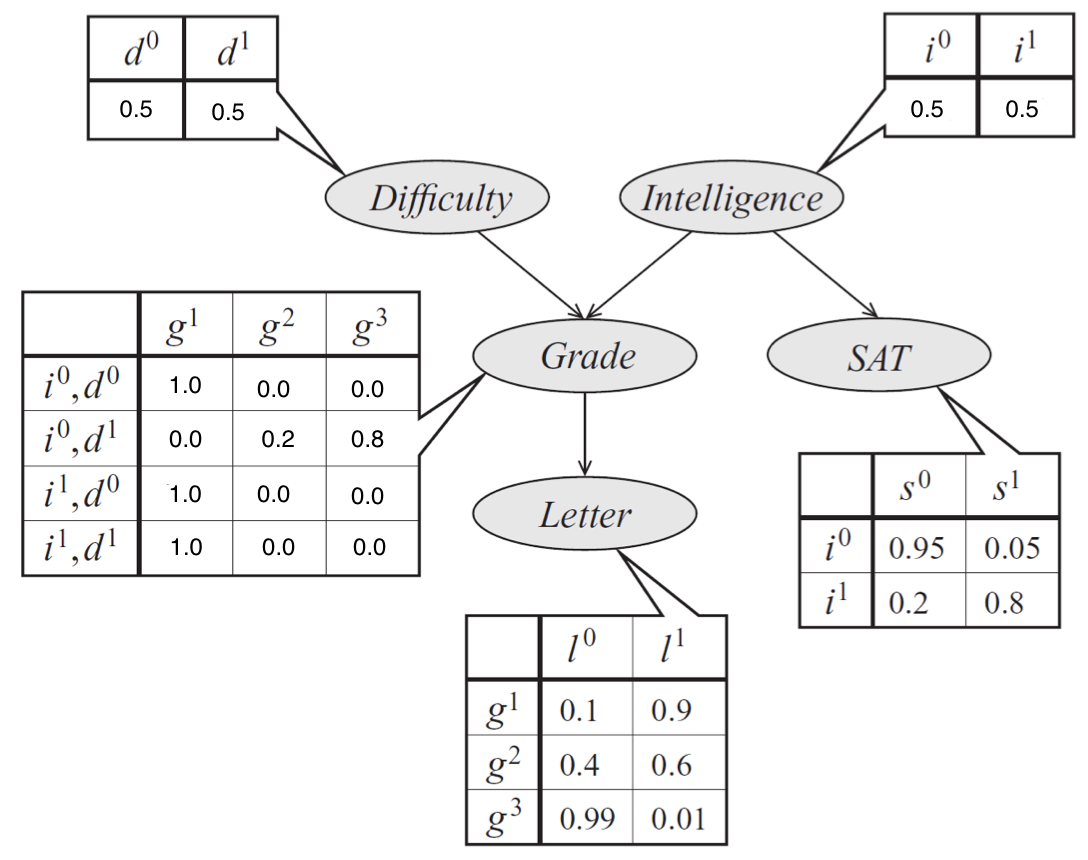a) Write the a table giving joint distribution of Difficulty $$D$$ and Intelligence $$I$$.

Solution

Variables $$I$$ and $$D$$ are marginally independent, so their joint distribution factorizes into $$P(I,D) = P(I)P(D)$$

I D P(I,D)
0 0 0.25
0 1 0.25
1 0 0.25
1 1 0.25

b) Write the table giving the joint conditional distribution of Difficulty $$D$$ and Intelligence $$I$$ given that Grade = 1.

Solution

Because they're leaf nodes, we can ignore Letter and SAT. The rest of the answer can be computed using the definition of conditional distributions.

c) What is the probability of Intelligence given that Grade = 1?

d) What is the probability of Intelligence given that Grade = 1 and Difficulty = 0?